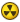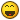## Recommended Posts

Ok guys, I want to convert a number to time format.

\$TimeLeft = Round(\$MinutesLeft, 1)

This is code will give me numbers like 1.5 minutes left. How can I convert that to 1:30 (Minutes:Seconds)

Thanks guys!

##### Share on other sites

can you show us the script... how you got \$timeleft

8)

##### Share on other sites

Ok guys, I want to convert a number to time format.

\$TimeLeft = Round(\$MinutesLeft, 1)

This is code will give me numbers like 1.5 minutes left. How can I convert that to 1:30 (Minutes:Seconds)

Thanks guys!

Just do this

```MsgBox(0, \$Timeleft, DecToTime(\$TimeLeft))

Func DecToTime(\$decimal)
\$Min_Sec = StringSplit(\$decimal, ".")
\$DecSecs = 10/\$Min_Sec
\$TimeSecs = 60/\$DecSecs
Return \$Min_Sec&":"&\$TimeSecs
EndFunc```
Edited by Paulie

##### Share on other sites

Here is my program code in full. It just automates a task and im trying to create almost a progress ticker for it.

```#include <GUIConstants.au3>
\$hMainGUI = GUICreate("Auto Publisher", 200, 100); save handle to GUI
\$Label_1 = GUICtrlCreateLabel("Publish how many?", 30, 10); save control ID of label (in case you want to change it later)
\$Input_1 = GUICtrlCreateInput("10", 30, 50, 60); save control ID of input (for reading it later)
\$Button_1 = GUICtrlCreateButton("Publish", 100, 47, 60); save control ID of button (to see if it's pushed)
GUISetState(@SW_SHOW)

While 1
\$Msg = GuiGetMsg()
Switch \$Msg
Case \$GUI_EVENT_CLOSE
ExitLoop; Exit loop if GUI gets closed

Case \$Button_1
MsgBox(64, "Auto Publisher", "Number to Publish:  " & \$InputData)
ExitLoop; Exit loop when done
EndSwitch
Wend

MsgBox(64, "Auto Publisher", "Make sure you have the first email open.")

For \$i = \$InputData to 1 Step -1

\$SecondsLeft = \$InputLeft * 9.25
\$MinutesLeft = \$SecondsLeft / 60
\$TimeLeft =  Round(\$MinutesLeft, 1)

\$Completed = \$TotalPosts - \$InputLeft
\$PercentComplete = \$Completed / \$TotalPosts

MouseClick("left", 343, 616, 2); Link to the publish page
Sleep(3000);two seconds
MouseClick("left", 55, 307, 2);Publish Button
Sleep(3000);two seconds
MouseClick("left", 1260, 12, 2);Close Window
Sleep(250);1/4 a second
MouseClick("left", 1236, 406, 2);Next Button
\$InputLeft = \$InputLeft - 1
#include <GUIConstants.au3>
GUICreate("Progress", 200, 80); save handle to GUI
GUICtrlCreateLabel(\$InputLeft &" left to publish.", 30, 10)
GUICtrlCreateLabel("About "& \$TimeLeft &" minutes left.", 30, 30)
GUICtrlCreateLabel(\$PercentComplete &"% complete.", 30, 50)
GUISetState(@SW_SHOW)

Sleep(3000);two seconds
Next
MsgBox(0,"", \$InputData & " posting(s) published.")```

##### Share on other sites

Ok guys, I want to convert a number to time format.

\$TimeLeft = Round(\$MinutesLeft, 1)

This is code will give me numbers like 1.5 minutes left. How can I convert that to 1:30 (Minutes:Seconds)

Thanks guys!

How about multiple the decimal part by 60 - that will give you 30 in your example:

30 = 0.5 * 60

All by me:

"Sometimes you have to go back to where you started, to get to where you want to go."

"Everybody catches up with everyone, eventually"

"As you teach others, you are really teaching yourself."

"Do not worry about yesterday, as the only thing that you can control is tomorrow."Programming Tips

Excel Changes

ControlHover.UDF

GDI_Plus

Draw_On_Screen

GDI Basics

GDI_More_Basics

GDI Rotate

GDI Graph

GDI  CheckExistingItems

GDI Trajectory

Replace \$ghGDIPDll with \$__g_hGDIPDll

DLL 101?

Array via Object

GDI Swimlane

GDI Plus French 101 Site

GDI Examples UEZ

GDI Basic Clock

GDI Detection

# Ternary operator

##### Share on other sites

How about multiple the decimal part by 60 - that will give you 30 in your example:

30 = 0.5 * 60

EDIT - that will make me read the post again before posting - I made something to eat and came back and just posted

All by me:

"Sometimes you have to go back to where you started, to get to where you want to go."

"Everybody catches up with everyone, eventually"

"As you teach others, you are really teaching yourself."

"Do not worry about yesterday, as the only thing that you can control is tomorrow."Programming Tips

Excel Changes

ControlHover.UDF

GDI_Plus

Draw_On_Screen

GDI Basics

GDI_More_Basics

GDI Rotate

GDI Graph

GDI  CheckExistingItems

GDI Trajectory

Replace \$ghGDIPDll with \$__g_hGDIPDll

DLL 101?

Array via Object

GDI Swimlane

GDI Plus French 101 Site

GDI Examples UEZ

GDI Basic Clock

GDI Detection

# Ternary operator

##### Share on other sites

How about multiple the decimal part by 60 - that will give you 30 in your example:

30 = 0.5 * 60*Points to post #3*Edited by Paulie

##### Share on other sites*Points to post #3*I know - I should have just posted my answer before trying to eat - but at least you gave an example - you get extra points for thatAll by me:

"Sometimes you have to go back to where you started, to get to where you want to go."

"Everybody catches up with everyone, eventually"

"As you teach others, you are really teaching yourself."

"Do not worry about yesterday, as the only thing that you can control is tomorrow."Programming Tips

Excel Changes

ControlHover.UDF

GDI_Plus

Draw_On_Screen

GDI Basics

GDI_More_Basics

GDI Rotate

GDI Graph

GDI  CheckExistingItems

GDI Trajectory

Replace \$ghGDIPDll with \$__g_hGDIPDll

DLL 101?

Array via Object

GDI Swimlane

GDI Plus French 101 Site

GDI Examples UEZ

GDI Basic Clock

GDI Detection

# Ternary operator

##### Share on other sites

is there any way to take the remainder from rounding?

##### Share on other sites

Try this?

```\$test1 = "00:50"
\$test2 = "00:40"

MsgBox(0,"Time", "\$test1 = " & \$test1 & @CRLF & "\$test2 = " & \$test2)
MsgBox(0,"Result Sub", _TimeSub(\$test1,\$test2)) ;Will return 00:10

\$aVal1 = StringSplit(\$iVal1,":")
If @error Then
SetError(1) ; Time in incorrect format
Return 0
EndIf
\$aVal2 = StringSplit(\$iVal2,":")
If @error Then
SetError(1) ; Time in incorrect format
Return 0
EndIf
If (\$aVal1 > 4) Or (\$aVal2 > 4) Then
SetError(1) ; Time in incorrect format
Return ""
ElseIf \$aVal1 = 2 Then
\$iHourA = 0
\$iMinA = \$aVal1
\$iSecA = \$aVal1
ElseIf \$aVal1 = 3 Then
\$iHourA = \$aVal1
\$iMinA = \$aVal1
\$iSecA = \$aVal1
EndIf
If \$aVal2 = 2 Then
\$iHourB = 0
\$iMinB = \$aVal2
\$iSecB = \$aVal2
ElseIf \$aVal2 = 3 Then
\$iHourB = \$aVal2
\$iMinB = \$aVal2
\$iSecB = \$aVal2
EndIf
If (\$iSecA + \$iSecB) < 60 Then
\$iSecC = (\$iSecA + \$iSecB)
ElseIf (\$iSecA + \$iSecB) > 59 Then
\$iSecC = (\$iSecA + \$iSecB) - 60
\$iMinA = \$iMinA + 1
EndIf
If (\$iMinA + \$iMinB) < 60 Then
\$iMinC = (\$iMinA + \$iMinB)
ElseIf (\$iMinA + \$iMinB) > 59 Then
\$iMinC = (\$iMinA + \$iMinB) - 60
\$iHourA = \$iHourA + 1
EndIf
\$iHourC = \$iHourA + \$iHourB
If StringLen(\$iSecC) = 1 Then \$iSecC = "0" & \$iSecC
If StringLen(\$iMinC) = 1 Then \$iMinC = "0" & \$iMinC
If \$iHourC = 0 Then
Return (\$iMinC & ":" & \$iSecC)
ElseIf \$iHourC <> 0 Then
Return (\$iHourC & ":" & \$iMinC & ":" & \$iSecC)
EndIf
EndFunc

Func _TimeSub(\$iVal1,\$iVal2)
\$aVal1 = StringSplit(\$iVal1,":")
If @error Then
SetError(1) ; Time in incorrect format
Return 0
EndIf
\$aVal2 = StringSplit(\$iVal2,":")
If @error Then
SetError(1) ; Time in incorrect format
Return 0
EndIf
If (\$aVal1 > 4) Or (\$aVal2 > 4) Then
SetError(1) ; Time in incorrect format
Return ""
ElseIf \$aVal1 = 2 Then
\$iHourA = 0
\$iMinA = \$aVal1
\$iSecA = \$aVal1
ElseIf \$aVal1 = 3 Then
\$iHourA = \$aVal1
\$iMinA = \$aVal1
\$iSecA = \$aVal1
EndIf
If \$aVal2 = 2 Then
\$iHourB = 0
\$iMinB = \$aVal2
\$iSecB = \$aVal2
ElseIf \$aVal2 = 3 Then
\$iHourB = \$aVal2
\$iMinB = \$aVal2
\$iSecB = \$aVal2
EndIf
If (\$iSecA - \$iSecB) > 0 Then
\$iSecC = (\$iSecA - \$iSecB)
ElseIf (\$iSecA - \$iSecB) = 0 Then
\$iSecC = "00"
ElseIf (\$iSecA - \$iSecB) < 1 Then
\$iSecC = 60 + (\$iSecA - \$iSecB)
\$iMinA = \$iMinA - 1
EndIf
If (\$iMinA - \$iMinB) > 0 Then
\$iMinC = (\$iMinA - \$iMinB)
ElseIf (\$iMinA - \$iMinB) = 0 Then
\$iMinC = "00"
ElseIf (\$iMinA - \$iMinB) < 1 Then
\$iMinC = 60 + (\$iMinA - \$iMinB)
\$iHourA = \$iHourA - 1
EndIf
\$iHourC = \$iHourA - \$iHourB
If StringLen(\$iSecC) = 1 Then \$iSecC = "0" & \$iSecC
If StringLen(\$iMinC) = 1 Then \$iMinC = "0" & \$iMinC
If \$iHourC = 0 Then
Return (\$iMinC & ":" & \$iSecC)
ElseIf \$iHourC <> 0 Then
Return (\$iHourC & ":" & \$iMinC & ":" & \$iSecC)
EndIf
EndFunc```

qq

## Create an account

Register a new account

• ### Recently Browsing   0 members

×

• Wiki

• Back

• #### Beta

• Git
• FAQ
• Our Picks
×
• Create New...